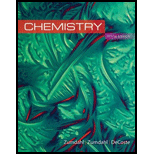Chemistry
10th Edition
ISBN: 9781305957404
Author: Steven S. Zumdahl, Susan A. Zumdahl, Donald J. DeCoste
Publisher: Cengage LearningChemistry
Stoichiometry. 125E

# The amount of hydrogen peroxide that can be produced by the given chemical reaction is to be calculated. The mass of the unreacted reagent is to be calculated. Concept introduction: The mass of a substance can be obtained by using the number of moles of the substance present and its molar mass. The formula used to calculate the mass of a given substance is, Mass of the substance = ( Number of moles ) × ( Molar mass of the substance ) To determine: The mass of hydrogen peroxide that can be produced by the given chemical reaction and the mass of the unreacted reagent.

QuestionChapter 3, Problem 125E
Interpretation Introduction

## Interpretation: The amount of hydrogen peroxide that can be produced by the given chemical reaction is to be calculated. The mass of the unreacted reagent is to be calculated.Concept introduction: The mass of a substance can be obtained by using the number of moles of the substance present and its molar mass. The formula used to calculate the mass of a given substance is, Mass of the substance=(Number of moles)×(Molar mass of the substance) To determine: The mass of hydrogen peroxide that can be produced by the given chemical reaction and the mass of the unreacted reagent.

The amount of hydrogen peroxide that can be produced is 0.301g_ . The mass of the unreacted reagent, that is HCl , is 3.6×10-2g_ .

### Explanation of Solution

To determine: The mass of hydrogen peroxide that can be produced by the given chemical reaction

Given

The stated chemical reaction is,

BaO2(s)+2HCl(aq)H2O2(aq)+BaCl2(aq)

The given mass of BaO2 is 1.50g .

The given volume of HCl is 25.0ml .

The solution contains 0.0272gHClperml .

The molar mass of BaO2 =Ba+2O=(137+(2×16))g/mol=169g/mol

Formula

The number of moles of a substance is calculated by the formula,

Numberofmoles=GivenmassofthesubstanceMolarmassofthesubstance

Substitute the value of the given mass and the molar mass of BaO2 in the above expression.

NumberofmolesofBaO2=1.50g169g/mol=8.87×103mol

The solution contains 0.0272gHClperml .

Therefore, the mass of HCl =(0.0272×25.0)g=0.68g

The molar mass of HCl =H+Cl=(1+35.5)g/mol=36.5g/mol

NumberofmolesofHCl=0.68g36.5g/mol=1.86×102mol

According to the stated reaction,

1mol of BaO2 reacts with 2mol of HCl .

8.87×103mol of BaO2 reacts with HCl =(2×8.87×103)mol=1.77×102mol

The moles of HCl given are 1.86×102mol .

Therefore, some amount of HCl is left unreacted. It is the excess reactant.

Barium oxide (BaO2) is the limiting reagent.

According to the stated reaction,

1mol of BaO2 produces 1mol of H2O2 .

8.87×103mol of BaO2 produces H2O2 =(1×8.87×103)mol=8.87×103mol

Molar mass of H2O2 =2O+2H=((2×16)+(2×1))g/mol=34g/mol

The mass of a substance is calculated by the formula,

Massofthesubstance=(Numberofmoles)×(Molarmassofthesubstance)

Substitute the value of the number of moles of H2O2 and the molar mass of H2O2 in the above expression.

MassofH2O2=(8.87×103mol)×(34g/mol)=0.301g_

To determine: The unreacted amount of the starting material.

Given

The stated chemical reaction is,

BaO2(s)+2HCl(aq)H2O2(aq)+BaCl2(aq)

The given mass of BaO2 is 1.50g .

The given volume of HCl is 25.0ml .

The solution contains 0.0272gHClperml .

The molar mass of BaO2 =Ba+2O=(137+(2×16))g/mol=169g/mol

Formula

The number of moles of a substance is calculated by the formula,

Numberofmoles=GivenmassofthesubstanceMolarmassofthesubstance

Substitute the value of the given mass and the molar mass of BaO2 in the above expression.

NumberofmolesofBaO2=1.50g169g/mol=8.87×103mol

According to the stated reaction,

1mol of BaO2 reacts with 2mol of HCl .

8.87×103mol of BaO2 reacts with HCl =(2×8.87×103)mol=1.77×102mol

The moles of HCl given are 1.86×102mol .

Therefore, some amount of HCl is left unreacted. It is the excess reactant.

The moles of HCl left unreacted are calculated by the formula,

UnreactedmolesofHCl=GivenmolesofHClReactedmolesofHCl

Substitute the values of the given moles of HCl and the reacted moles of HCl in the above expression.

UnreactedmolesofHCl=(1.86×1021.77×102)mol=0.09×102mol

Molar mass of HCl =H+Cl=(1+35.5)g/mol=36.5g/mol

The mass of a substance is calculated by the formula,

Massofthesubstance=(Numberofmoles)×(Molarmassofthesubstance)

Substitute the value of the number of moles of HCl and the molar mass of HCl in the above expression.

MassofHCl=(0.09×102mol)×(36.5g/mol)=3.3×10-2g_

Conclusion

The amount of hydrogen peroxide that can be produced is 0.301g_ .

The unreacted reagent is HCl and the unreacted mass of HCl is 3.6×10-2g_ .

### Want to see more full solutions like this?

Subscribe now to access step-by-step solutions to millions of textbook problems written by subject matter experts!Chemistry
10th Edition
ISBN: 9781305957404
Author: Steven S. Zumdahl, Susan A. Zumdahl, Donald J. DeCoste
Publisher: Cengage LearningChemistry
Stoichiometry. 125ESimilar questions
To this solutionStoichiometryMore videos on
StoichiometryWant to see more full solutions like this?
Subscribe now to access step-by-step solutions to millions of textbook problems written by subject matter experts.
Knowledge BoosterNeed a deep-dive on the concept behind this application? Watch these videos for an in-depth look
Bonding (Ionic, Covalent & Metallic) - GCSE Chemistry
Science Shorts
Stoichiometry - Chemistry for Massive Creatures: Crash Course Chemistry #6
Crash Course
Recommended textbooks for you
•Chemistry
Chemistry
ISBN:9781305957404
Author:Steven S. Zumdahl, Susan A. Zumdahl, Donald J. DeCoste
Publisher:Cengage LearningChemistry
Chemistry
ISBN:9781133611097
Author:Steven S. Zumdahl
Publisher:Cengage LearningChemistry: An Atoms First Approach
Chemistry
ISBN:9781305079243
Author:Steven S. Zumdahl, Susan A. Zumdahl
Publisher:Cengage Learning
•Living By Chemistry: First Edition Textbook
Chemistry
ISBN:9781559539418
Author:Angelica Stacy
Publisher:MAC HIGHERGeneral Chemistry - Standalone book (MindTap Cour...
Chemistry
ISBN:9781305580343
Author:Steven D. Gammon, Ebbing, Darrell Ebbing, Steven D., Darrell; Gammon, Darrell Ebbing; Steven D. Gammon, Darrell D.; Gammon, Ebbing; Steven D. Gammon; Darrell
Publisher:Cengage LearningChemistry for Engineering Students
Chemistry
ISBN:9781337398909
Author:Lawrence S. Brown, Tom Holme
Publisher:Cengage Learning
•Chemistry
Chemistry
ISBN:9781305957404
Author:Steven S. Zumdahl, Susan A. Zumdahl, Donald J. DeCoste
Publisher:Cengage LearningChemistry
Chemistry
ISBN:9781133611097
Author:Steven S. Zumdahl
Publisher:Cengage LearningChemistry: An Atoms First Approach
Chemistry
ISBN:9781305079243
Author:Steven S. Zumdahl, Susan A. Zumdahl
Publisher:Cengage LearningLiving By Chemistry: First Edition Textbook
Chemistry
ISBN:9781559539418
Author:Angelica Stacy
Publisher:MAC HIGHERGeneral Chemistry - Standalone book (MindTap Cour...
Chemistry
ISBN:9781305580343
Author:Steven D. Gammon, Ebbing, Darrell Ebbing, Steven D., Darrell; Gammon, Darrell Ebbing; Steven D. Gammon, Darrell D.; Gammon, Ebbing; Steven D. Gammon; Darrell
Publisher:Cengage LearningChemistry for Engineering Students
Chemistry
ISBN:9781337398909
Author:Lawrence S. Brown, Tom Holme
Publisher:Cengage Learning